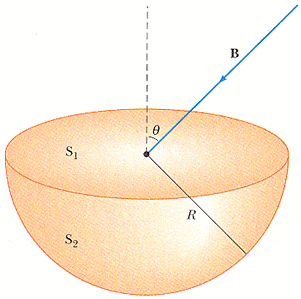# Magnetic Flux through a hemisphere.

Slimmy

## Homework Statement

Symbolic question:
Consider the hemispherical closed surface in Figure P30.34. The hemisphere is in a uniform magnetic field that makes an angle θ with the vertical.(a) Calculate the magnetic flux through the flat surface (S1), using pi for π, theta for θ, B, and R as necessary.
(b) Calculate the magnetic flux through the hemispherical surface (S2), using pi for π, theta for θ, B, and R as necessary.

## Homework Equations

Magnetic Flux =
[Surface integral](B (dot) dA)
(My apologies for...not knowing how to insert equations yet)

## The Attempt at a Solution

Well, the problem states the magnetic field is uniform, so, at least for the flat surface, I should be able to pull the "B" out of the integral, as well as the cos(theta) so, you are left with Bcos(theta)piR^(2), (the area of surface one). Yet it is incorrect. For part b, I would assume it would be the same as part a, seeing as how it is a surface integral. (If my concept of surface integrals are correct)

Last edited by a moderator:

nickjer
Please make separate posts for those 2 problems. Your solution to (a) is right. If this is some sort of web based homework, then you might want to check that everything is typed in correctly.

For part (b) you can use Gauss's law for magnetism which states that the total magnetic flux through a closed surface must be 0.

Slimmy
Please make separate posts for those 2 problems. Your solution to (a) is right. If this is some sort of web based homework, then you might want to check that everything is typed in correctly.

For part (b) you can use Gauss's law for magnetism which states that the total magnetic flux through a closed surface must be 0.
My apology for that.

Well, for part (a) I have tried my answer, yet, it is still wrong, and yes I have checked to make sure it was typed in correctly.
For part (b) I believe it only wants the flux for the surface of two, so it is not closed without surface one, because zero was the incorrect answer as well.

nickjer
The total closed surface is the sum of the two surfaces. So the sum of the fluxes should be 0. Since you know the flux through surface (1), then you can easily get the flux through surface (2).

Slimmy
Ah, so the magnetic flux for the second surface is just the negative of the first surface? Yet, if that is the case...I still cannot obtain the correct answer for part (a).
This is exactly what I typed in the answer box.
cos(theta)*B*pi*R^2
And I looked at the preview of the answer..and everything seems to be okay.
It is still incorrect.

nickjer
Maybe set the first answer negative, and the 2nd answer positive. Other than that, I am out of ideas.

Slimmy
Yup, you were right, I had to switch them...yet, I am still clueless as to why... But thank you for your help!

nickjer
Doesn't matter too much. Some people prefer flux into a closed surface to be negative, others positive. Seems your book prefers flux into a closed surface to be negative.